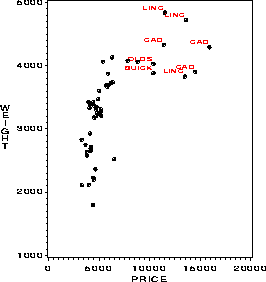label Annotate dataset to label observations in a plot label

# SAS Macro Programs: label

\$Version: 1.8 (11 Nov 2008)
Michael Friendly
York University

## The label macro (get label.sas)

### Create an Annotate dataset to label observations in a plot

label is a utility macro which creates an Annotate dataset to label observations in a 2D (PROC GPLOT) or 3D (PROC G3D) scatterplot. The points which are labelled may be selected by an arbitrary logical expression from those in the input dataset. The resulting Annotate dataset would then be used with the ANNO= option of PROC GPLOT or PROC G3D.

## Usage

label is a macro program. Values must be supplied for the X= and Y= parameters. For a G3D plot, supply a value for the Z= parameter as well.

The arguments may be listed within parentheses in any order, separated by commas. The Annotate dataset is then used with PROC GPLOT. For example:

```   %label(data=inputdataset, x=xvar, y=yvar, ..., )
proc gplot data=inputdataset;
plot yvar * xvar /  anno=_LABEL_ ... ;
```

### Parameters

X=
X variable for scatterplot
Y=
Y variable for scatterplot
Z=
Z variable for G3D (optional)
XOFF=0
X-offset for label. The offset may be specified as a numeric constant or as the name of a variable in the data set. Positive values move the label to the right relative to the point.
YOFF=0
Y-offset for label. Positive values move the label upwards relative to the point.
ZOFF=0
Z-offset for label
TEXT=
text used to label each point. TEXT may be specified as a variable in the data set or SAS expression involving dataset variables and/or string constants. If you supply an expression, use the `%str()` macro function, e.g., `TEXT=%str(name || '-' || place)` to protect special characters.
LEN=8
length of TEXT variable
POS=
Position for label relative to the data point. The POS= value can be a character constant (one of the characters in "123456789ABCDEF<+>", as used by the Annotate POSITION variable), an expression involving dataset variables which evaluates to one of these characters, or one of the special characters, "/", "|", or "-". The special position values cause the point label to be out-justified (moved outward toward the edges of the plot relative to the data point.) by comparing the coordinates of the point to the mean of X and Y (/), or to the mean of X only (|), or to the mean of Y only (-).
SYS=2
XSYS & YSYS value
COLOR='BLACK'
label color: a dataset variable or string constant enclosed in quotes.
SIZE=1
size of the label text
FONT=
The name of the font used for the label. There is no default, which means that the labels inherit the global FTEXT= setting.
SUBSET=1
An expression (which may involve any dataset variables) to select points. A point will be labelled if the expression evaluates to non-zero for the current observation.
OUT=_LABEL_
The name of the Annotate data set produced

### Example

This example plots Weight against Price for American cars in the Auto data, labelling the most expensive cars.
```%include macros(label);        *-- or include in an autocall library;
%label(data=auto, x=price, y=weight,
color='red', size=1.2,
subset=origin='A' and price>10000,
pos=1, text=scan(model,1));

proc gplot data=auto(where=(origin='A'));
plot weight * price / frame anno=_label_;
symbol1 v='+'  i=none color=black h=1.5;
run;
```### See also

boxanno Annotate a scatter plot with univariate boxplots
cpplot Plots of Mallow's C(p) and related statistics for model selection
outlier Robust multivariate outlier detection